# Measurement Scales of Measurement Stanley S Stevens Five

• Slides: 31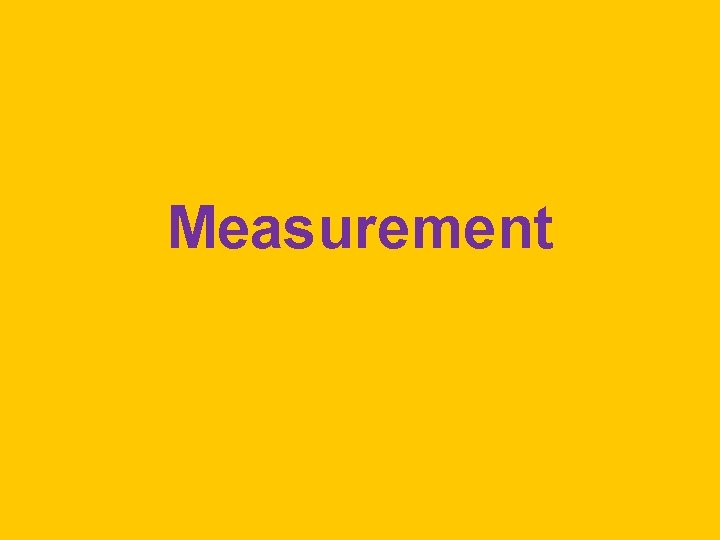MeasurementScales of Measurement • Stanley S. Stevens’ Five Criteria for Four Scales • Nominal Scales – 1. numbers are assigned to objects according to rules – can establish equivalence or nonequivalence of measured objectsOrdinal Scales 2. m(O 1 ) m(O 2 ) only if t(O 1 ) t(O 2 ) 3. m(O 1 ) > m(O 2 ) only if t(O 1 ) > t(O 2 )– equivalence or nonequivalence – order: which has more of the measured attribute – cannot establish equivalence of differencesInterval Scales • • • letting Xi stand for t(Oi ) 4. m(Oi ) = a + b. Xi, b > 0 t(O 1 ) ‑ t(O 2 ) = t(O 3 ) ‑ t(O 4 ) if m(O 1 ) ‑ m(O 2 ) = m(O 3 ) ‑ m(O 4 )Ratio Scales • • 5. a = 0, that is m(Oi ) = b. Xi, true zero point m(O 1 ) m(O 2 ) = b. X 1 b. X 2 = X 1 X 2 Remember gas law problems?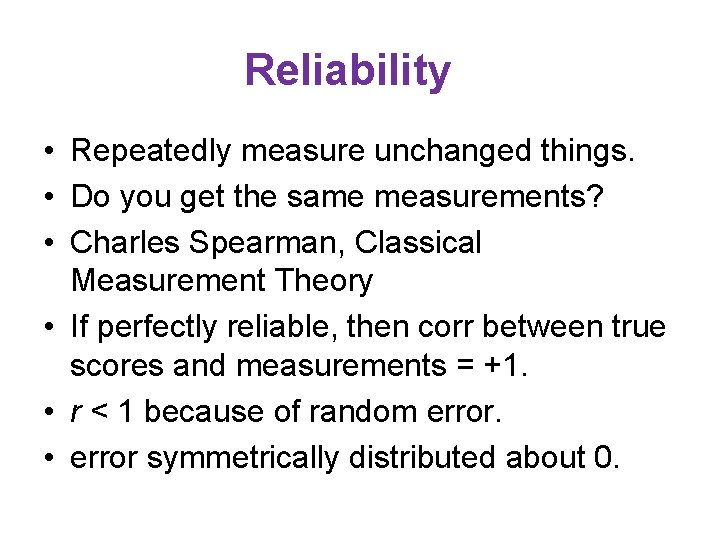Reliability • Repeatedly measure unchanged things. • Do you get the same measurements? • Charles Spearman, Classical Measurement Theory • If perfectly reliable, then corr between true scores and measurements = +1. • r < 1 because of random error. • error symmetrically distributed about 0.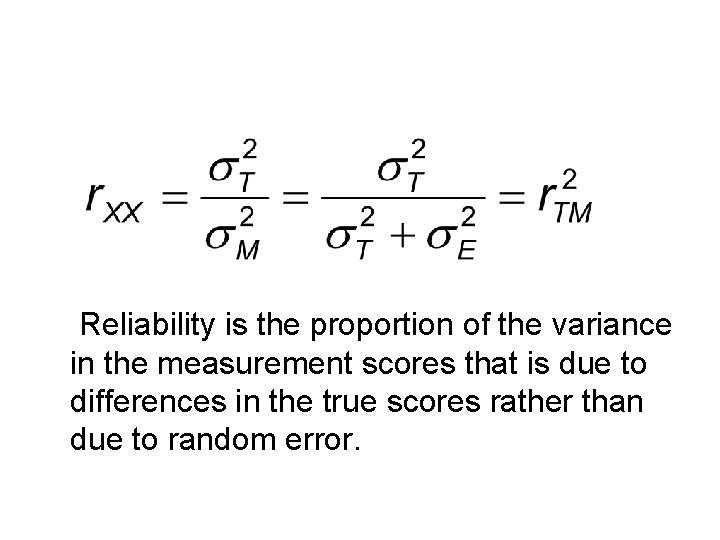Reliability is the proportion of the variance in the measurement scores that is due to differences in the true scores rather than due to random error.• Systematic error – not random – measuring something else, in addition to the construct of interest • Reliability cannot be known, can be estimated.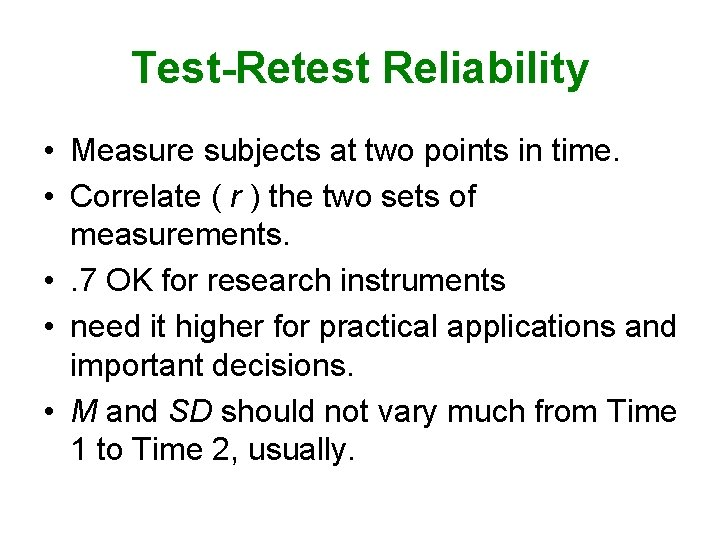Test-Retest Reliability • Measure subjects at two points in time. • Correlate ( r ) the two sets of measurements. • . 7 OK for research instruments • need it higher for practical applications and important decisions. • M and SD should not vary much from Time 1 to Time 2, usually.Alternate/Parallel Forms • Estimate reliability with r between forms. • M and SD should be same for both forms. • Pattern of corrs with other variables should be same for both forms.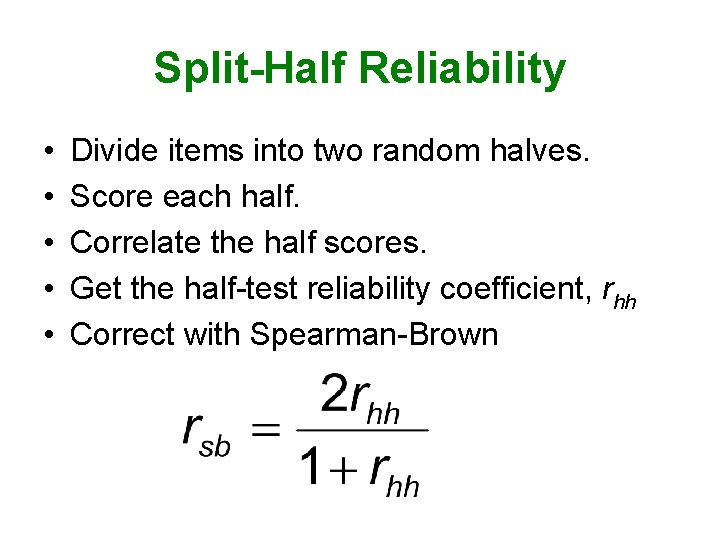Split-Half Reliability • • • Divide items into two random halves. Score each half. Correlate the half scores. Get the half-test reliability coefficient, rhh Correct with Spearman-BrownCronbach’s Coefficient Alpha • Obtained value of rsb depends on how you split the items into haves. • Find rsb for all possible pairs of split halves. • Compute mean of these. • But you don’t really compute it this way. • This is a lower bound for the true reliability. • That is, it underestimates true reliability.Maximized Lambda 4 • This is the best estimator of reliability. • Compute rsb for all possible pairs of split halves. • The largest rsb = the estimated reliability. • If more than a few items, this is unreasonably tedious. • But there are ways to estimate it.Intra-Rater Reliability • A single person is the measuring instrument. • Rate unchanged things twice. • Correlate the ratings.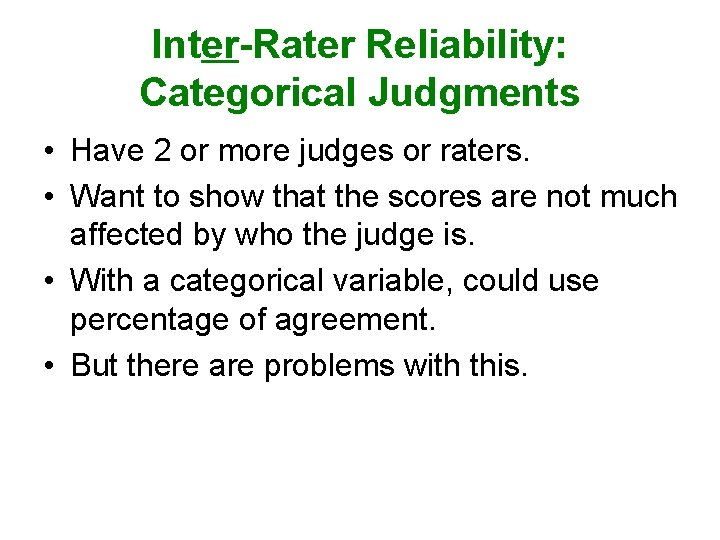Inter-Rater Reliability: Categorical Judgments • Have 2 or more judges or raters. • Want to show that the scores are not much affected by who the judge is. • With a categorical variable, could use percentage of agreement. • But there are problems with this.• % agreement = 80% • agree on whether is fighting or not • but not on whether is aggressor or victimCohen’s Kappa • Corrects for tendency to get high % just because one category is very often endorsed by both judges. • For each cell in main diagonal, compute E – E = (row total)(column total) / table total – upper left cell, E = 73(75) / 100 = 54. 75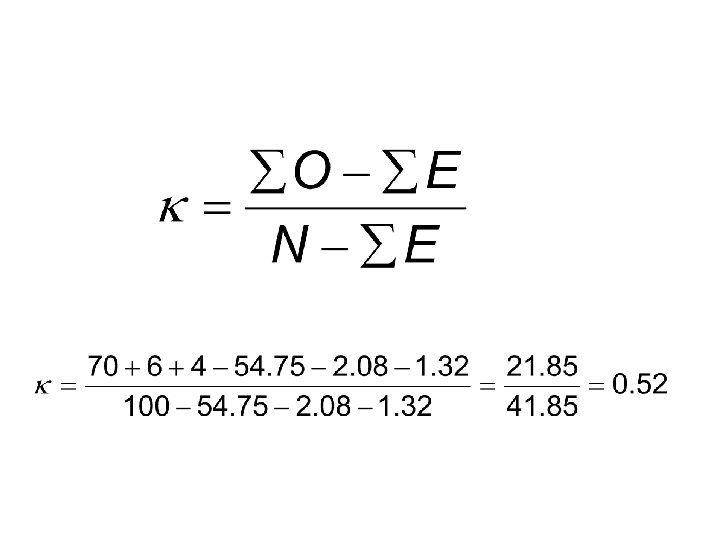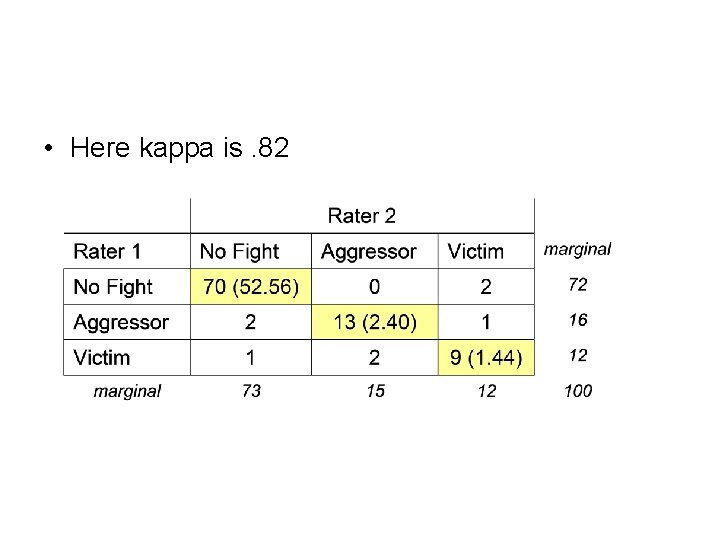• Here kappa is. 82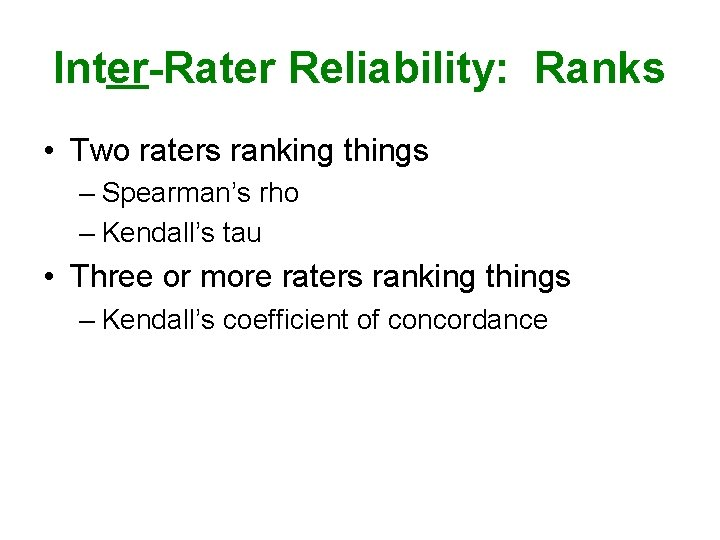Inter-Rater Reliability: Ranks • Two raters ranking things – Spearman’s rho – Kendall’s tau • Three or more raters ranking things – Kendall’s coefficient of concordanceInter-Rater Reliability: Continuous Scores • Two raters – could use Pearson r • Two or more raters – better to use intraclass correlation coefficient – scores could be highly correlated and show good agreementInter-Rater Reliability: Continuous Scores – or scores could be highly correlated but show little agreement – r =. 964 for both pairs of judges. – ICC =. 967 for first pair, . 054 for second pairConstruct Validity • To what extent are we really measuring/manipulating the construct of interest? • Face Validity – do others agree that it sounds valid?Content Validity • Detail the population of things (behaviors, attitudes, etc. ) that are of interest. • Consider our operationalization of the construct as a sample of that population. • Is our sample representative of the population – ask experts.Criterion-Related Validity • Established by demonstrating that our operationalization has the expected pattern of correlations with other variables. • Concurrent Validity – demonstrate the expected correlation with other variables measured at the same time. • Predictive Validity – demonstrate the expected correlation with other variables measured later in time.• Convergent Validity – demonstrate the expected correlation with measures of other constructs. • Discriminant Validity – demonstrate the expected lack of correlation with measures of other constructs.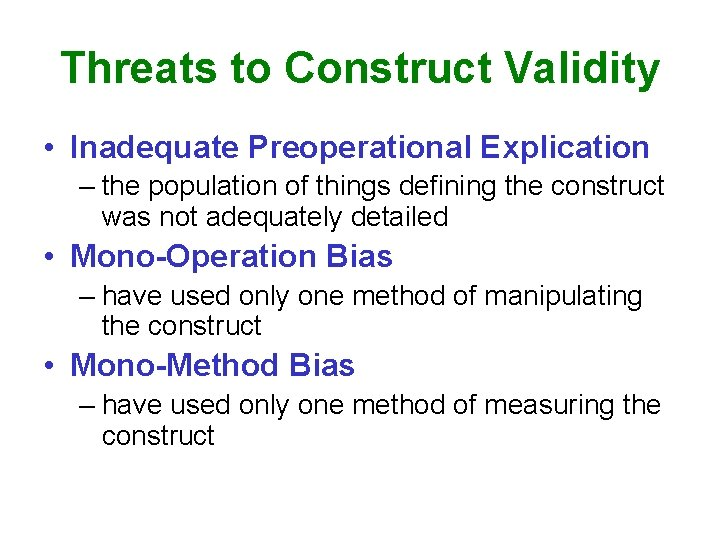Threats to Construct Validity • Inadequate Preoperational Explication – the population of things defining the construct was not adequately detailed • Mono-Operation Bias – have used only one method of manipulating the construct • Mono-Method Bias – have used only one method of measuring the construct• Interaction of Different Treatments – effect of manipulation of one construct is altered by the previous manipulation of another construct • Testing x Treatment Interaction – in pretest posttest design, did taking the pretest alter the effect of the treatment?• Restricted Generalizability Across Constructs – experimental treatment might affect constructs we did not measure – so we can’t describe the full effects of the treatment • Confounding Constructs with Levels of Constructs – Would our manipulated construct have a different effect if we used different levels of it?• Social Threats – Hypothesis Guessing • Good guy effect • Screw you effect – Evaluation Apprehension – Expectancy Effects • Experimenter expectancy • Participant expectancy • Blinding and double blinding